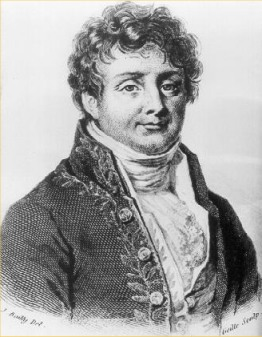In the eighteenth century, the most influential theory of what heat was and how it worked was a partially quantified theory of heat substance, called "caloric." Siméon-Denis Poisson, Antoine Lavoisier, and others of the Laplacian school developed precise experimental methods for measuring the amount of caloric a given substance contained.By 1800, alternatives to the caloric hypothesis appeared. Between 1807 and 1811, Joseph Fourier (1768-1830) developed a mathematical theory of heat conduction that was entirely independent of the caloric hypothesis, but the theory was not published until 1822, when it appeared as Théorie analytique de la chaleur (The Analytical Theory of Heat). Fourier's first step was to avoid speculation about "caloric." In this way, Fourier set the study of the theory of heat in the tradition of rational mechanics, basing it on differential equations that characterized the transmission of heat, equations that were independent of all physical hypotheses. In contrast to Poisson (who was, as mentioned above, a devoted Laplacian, committed to physical mechanics and to the existence of caloric), Fourier focused on heat flow, using differential equations to express how much heat diffused from a substance over time. The heat transmitted between two molecules was proportional to the difference in their temperature and a function of the distance between them, which of course varied with the nature of the intervening substance. Though formally (that is, mathematically) equivalent to Poisson's model, Fourier did not rely upon any speculation about the nature of heat. For Fourier, what was important was not what heat was, but what it did, in a given experimental setting.

Others considered heat in terms of its relation to work, and especially in terms of steam engine efficiency. Sadi Carnot, for example, observed that heat cannot flow directly between contiguous parts of a steam engine that are at different temperatures. From this observation, he concluded that the maximum theoretical efficiency of a steam engine depends only on temperature differentials between the heat reservoirs between which it runs, and not on the nature of the gas inside it. In a different way, James Prescott Joule also considered heat and work, arguing that the work developed by a heat engine is obtained from the very destruction of heat — in other words, heat and work have a reciprocal relationship in which energy is conserved.

In 1855, William Thomson and William Rankine synthesized this earlier work in proclaiming a new science of thermodynamics or "energetics," founded on two principles derived from the combined work of Joule and Carnot: the conservation of energy, and its dissipation, or entropy. The connection between energy and mechanical work was made clear, as was the distinction between potential and kinetic energy; work, power, and entropy were precisely defined. The power of an energy-based view of physics was formidable; some, such as Wilhelm Ostvald, claimed that energy was the sole real substance in nature.